Next: Variational Equations Up: Nuclear Energy Density Functional Previous: The Densities

## The Energy Density Functional

The energy density functional has the form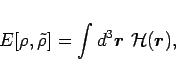(3)

where energy density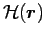is usually written as a sum of the p-h energy density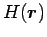and the p-p energy density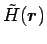. According to the DFT, there exists a nuclear universal energy functional that yields the exact binding energy of the nuclear system. This functional includes, in principle, all many-nucleon correlations.

The actual form of the nuclear energy functional is unknown. The strategy adopted by many practitioners is to build a functional around that generated by the Skyrme interaction. The most general form of the energy density functional that is quadratic in local densities and preserves the basic symmetries of the strong interaction, such as time-reversal symmetry, charge invariance, and proton-neutron symmetry, has been proposed in Ref. . In practical applications, however, simplified forms of this functional have been used. For instance, one particular representation of the energy functional for the ground states of even-even nuclei can be written as::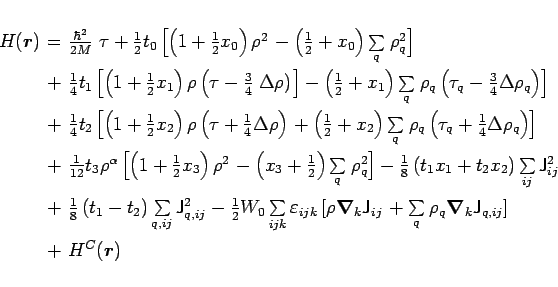(4)

and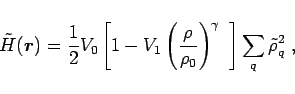(5)

where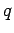labels the neutron (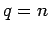) or proton (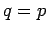) densities and the quantities which do not carry indexare the isoscalar densities (sums of proton and neutron densities; e.g.,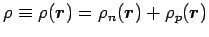).

The p-p energy functional (5) corresponds to a density-dependent delta interaction. Usually,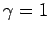,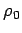=0.16 fm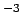, and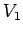=0, 1, or 1/2 for volume-, surface-, or mixed-type pairing. In Eq. (4),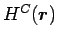stands for the Coulomb energy density with the exchange term treated in the Slater approximation.

As seen from Eqs. (4) and (5), typical Skyrme density functionals include about 14 unknown parameters. Some of them are usually adjusted to reproduce the basic properties of the infinite nuclear matter while the remaining coupling constants are fitted to known nuclear masses, radii, and other measured properties.Next: Variational Equations Up: Nuclear Energy Density Functional Previous: The Densities
Jacek Dobaczewski 2006-01-17